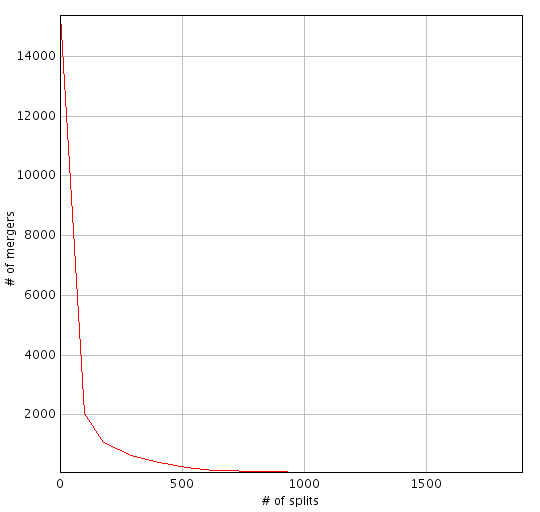# Minimum Splits and Mergers Warping errorThe content of this page has not been vetted since shifting away from MediaWiki. If you’d like to help, check out the how to help guide!Figure 1: example of splits vs mergers curve detected using the warping error metric.

When segmenting images with multiple objects, one might decide that some errors are not relevant compared to others. One of the advantages of the warping error is that it allows us to focus on only some desired types of topological errors1.

Here we propose a metric that takes only into account the number of splits and mergers produced while comparing two different labelings.

Given a set of original (binary) labels and its corresponding proposed (grayscale, i.e., probability map) labels, we can display the number of splits and mergers as a function of the threshold used to binarize the proposed labels (see Figure 1).

In the classic warping error, all pixels belonging to a topological error add to the final metric value. To make the result more intuitive, one can filter those pixels and select only the ones in which we are interested on, in our case, splits and mergers. This way, the metric value will correspond to the number of pixels of each split and merger divided by the total number of pixels. In other words, the metric represents the number of pixels that are needed to correct the segmentation.

## 2D implementation in Fiji

The minimum splits and mergers warping error metric is implemented for 2D images in the Trainable Weka Segmentation library. Here is an example of how to use it in Beanshell script:

``````import trainableSegmentation.metrics.WarpingError;

// original labels
originalLabels = IJ.openImage("/path/original-labels.tif");

// proposed (new) labels
proposedLabels = IJ.openImage("/path/proposed-labels.tif");

// assign original labels and proposal to the metric
metric = new WarpingError( originalLabels, proposedLabels );

// calculate metric for thresholds 0.0 to 0.9, in steps of 0.1
IJ.log("\nCalculating warping error by minimizing splits and mergers...");
metric = new WarpingError( originalLabels, proposedLabels );
warpingError = metric.getMinimumSplitsAndMergersErrorValue( 0.0, 0.9, 0.1, false );

// print results
IJ.log("  Warping error = " + warpingError);
IJ.log("  # errors (splits + mergers pixels) = " + Math.round(warpingError * originalLabels.getWidth() * originalLabels.getHeight() * originalLabels.getImageStackSize() ) );
``````